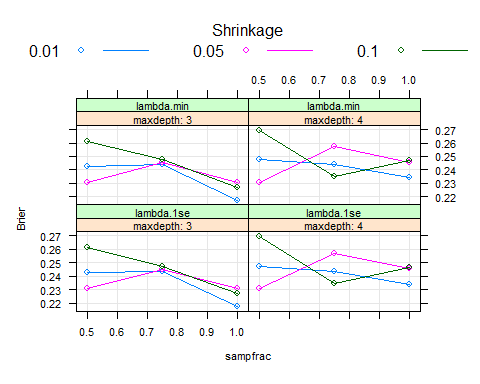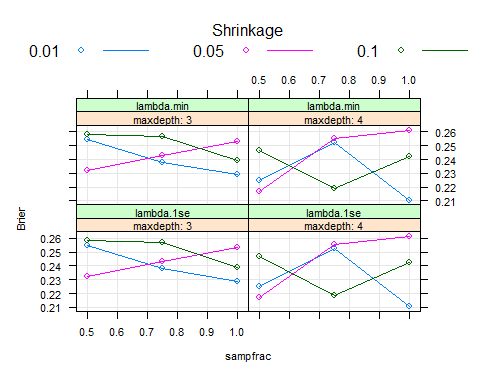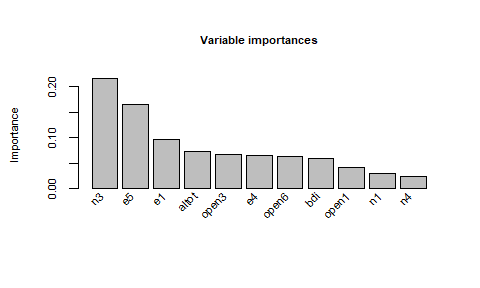# Tuning the parameters of function pre

## Introduction

Function pre() has a substantial number of model-fitting parameters, which may be tuned so as to optimize predictive accuracy and/or interpretability (sparsity) of the final model. For many of the parameters, default settings will likely perform well. Here, we discuss the effects of several of the parameters on predictive accuracy and model complexity. Next, we illustrate how to optimize predictive accuracy for a binary classification problem using package caret. We do not explain each argument in detail; readers are referred to the documentation of function pre() for that, or Fokkema (2020).

## Tuning parameters

The following arguments of function pre() can be expected to affect predictive accuracy and complexity of the final ensemble (ordered roughly from most to least likely candidates to require tuning):

• learnrate: Carefully tuning this parameter is likely to be the most beneficial in terms of predictive accuracy. There are no rules of thumb on how complexity and predictive accuracy will be affected by setting this argument to higher or lower than the default of 0.01. In light of the default value of ntrees = 500, the default of 0.01 is likely a very good starting points. Somewhat higher and lower values may be tried; lower values are likely beneficial only when argument ntrees is set to a higher value. A reasonable starting point would be to specify a grid search over c(0.01, 0.05, 0.1) or c(0.005, 0.01, 0.025, 0.05, 0.1).

• maxdepth: The default of three generates rules with at most 3 conditions, which are relatively easy to interpret. Somewhat higher or lower values may be preferred. Setting maxdepth = 1 will yield a model with main effects of predictor variables only.

• sampfrac: Depending on training sample size, values higher or lower than the default of .5 may be beneficial. Often smaller samples require higher values of sampfrac, sometimes even a value of 1 can be most beneficial for predictive accuracy; for larger samples, lower sampfrac values may suffice (and will reduce computation time).

• ntrees: Setting higher values than the default of 500 may increase complexity as well as predictive accuracy.

• type: The default of "both" will likely perform best in terms of predictive accuracy. Setting type = "rules" will yield a more complex final ensemble in most cases, but is unlikely to improve predictive accuracy. Setting type = "linear" simply yields a penalized regression model, which will likely have somewhat lower predictive accuracy, but also lower complexity.

• mtry: It is difficult to predict how setting this parameter to lower values than the default of mtry = Inf will affect complexity and predictive accuracy. Setting mtry to values $$< p$$ (the number of possible predictors) employs a random-forest like approach to rule induction. In the authors experience, the default boosting approach to rule induction works very well. Setting mtry to values $$< p$$ will however reduce the computational burden of rule generation, which may be desirable especially with larger datasets (large $$N$$ and/or large $$p$$).

• use.grad: the default of TRUE will likely perform well in most cases. With not-too-large sample sizes, setting use.grad = FALSE likely yields a more complex ensemble, which may have somewhat better predictive accuracy.

Furthermore, most methods for class pre (e.g., predict, print, summary, coef) take argument penalty.par.val, which by default is set to lambda.1se, as it tends to yield a good balance between predictive accuracy and complexity. Setting this argument to lambda.min may improve predictive accuracy but will also increase complexity of the final ensemble.

## Tuning parameters using package caret

We first load the required libraries:

library("caret")
library("pre")
library("pROC")
library("ggplot2")

The parameters of function pre() can be tuned using function train() from package caret. By default, squared error loss is minimized in case of numeric outcomes, which will be appropriate in many cases. (Weighted) misclassification error will be minimized by default for classification problems, which in most cases is not appropriate. In general, one should care about the quality of predicted probabilities, not just about the accuracy of the class labels assigned. Squared error loss on predicted probabilities (also known as the Brier score) should in many cases be preferred, or perhaps the area under the receiver operating curve.

To adjust the default of optimizing classification error, we set up a custom function in order to optimize the Brier score or AUC. For numeric outcomes, this will not be necessary, and the summaryFunction of the trainControl function need not be specified (see next code chunk).

BigSummary <- function (data, lev = NULL, model = NULL) {
brscore <- try(mean((data[, lev] - ifelse(data$obs == lev, 1, 0)) ^ 2), silent = TRUE) rocObject <- try(pROC::roc(ifelse(data$obs == lev, 1, 0), data[, lev],
direction = "<", quiet = TRUE), silent = TRUE)
if (inherits(brscore, "try-error")) brscore <- NA
rocAUC <- if (inherits(rocObject, "try-error")) {
NA
} else {
rocObject$auc } return(c(AUCROC = rocAUC, Brier = brscore)) } Next, we set up a control object for the train() function. Opinions may vary on what are the best setting for optimizing tuning parameters on a training dataset. Here, we take a 10-fold cross validation approach. Often, 10 repeats of 10-fold cross validation should be preferred, to make results less dependent on a single choice of folds. This can be done by setting repeats = 10 instead of the default below: fitControl <- trainControl(method = "repeatedcv", number = 10, repeats = 1, classProbs = TRUE, ## get probabilities, not class labels summaryFunction = BigSummary, verboseIter = TRUE) Next, we set up a custom tuning grid for the parameters of function pre(): preGrid <- getModelInfo("pre")[]$grid(
maxdepth = 3L:4L,
learnrate = c(.01, .05, .1),
penalty.par.val = c("lambda.1se", "lambda.min"),
sampfrac = c(0.5, 0.75, 1.0))
head(preGrid)
##   sampfrac maxdepth learnrate mtry use.grad penalty.par.val
## 1     0.50        3      0.01  Inf     TRUE      lambda.1se
## 2     0.75        3      0.01  Inf     TRUE      lambda.1se
## 3     1.00        3      0.01  Inf     TRUE      lambda.1se
## 4     0.50        4      0.01  Inf     TRUE      lambda.1se
## 5     0.75        4      0.01  Inf     TRUE      lambda.1se
## 6     1.00        4      0.01  Inf     TRUE      lambda.1se

Note that tuning and fitting PREs can be computationally heavy, especially with increasing size of the tuning grid, and a smaller grid of tuning parameter values may be preferred.

Note that the ntrees parameter is not included in the default grid-generating function (getModelInfo("pre")[]$grid). One can deviate from the default of ntrees = 500 by passing this argument calling function train() multiple times, and specifying a different value for ntrees each time (example provided below). The same goes for other arguments of function pre() that are not part of the default tuning parameters of caret’s method "pre". In this example, we focus on the (perhaps somewhat uninteresting, but useful as an example) problem of predicting sex sexo from the carrillo data included in package pre (type ?carrillo for explanation of the data and variables). We rename the levels of the outcome variable because the train function of caret does not appreciate when factor levels are numbers: carrillo$sexo <- factor(paste0("g", as.character(carrillo$sexo))) Note that the current dataset is rather small (112 observations). Still, to illustrate the principle of tuning parameters using training data, and evaluating predictive accuracy of the final fitted model using unseen test observations, we make a 75-25% train-test split: set.seed(42) train_ids <- sample(1:nrow(carrillo), .75*nrow(carrillo)) Next, we optimize the parameters. Note that this is a computationally heavy task so we need to be patient. We specified verboseIter = TRUE above, so progress information will be printed to the command line: set.seed(42) pre_tune <- train(sexo ~ ., data = carrillo[train_ids, ], method = "pre", ntrees = 500, family = "binomial", trControl = fitControl, tuneGrid = preGrid, metric = "Brier", ## Specify "AUCROC" for optimizing AUC maximize = TRUE) set.seed(42) pre_tune2 <- train(sexo ~ ., data = carrillo[train_ids, ], method = "pre", ntrees = 1000, family = "binomial", trControl = fitControl, tuneGrid = preGrid, metric = "Brier", maximize = TRUE) Some warnings (Warning: from glmnet Fortran code (error code -83)) will be reported, but these are not worrying; for some models, computations for some values in the $$\lambda$$ path could not be completed. We inspect the results: ids <- which(pre_tune$results$Brier == min(pre_tune$results$Brier)) pre_tune$results[ids, c(1:6, 10)]
##    sampfrac maxdepth learnrate mtry use.grad penalty.par.val   BrierSD
## 25        1        3      0.01  Inf     TRUE      lambda.1se 0.1340773
## 26        1        3      0.01  Inf     TRUE      lambda.min 0.1340773
plot(pre_tune,
xlab = list(cex = .7), ylab = list(cex = .7),
scales = list(cex=.7),
par.strip.text=list(cex=.7))ids2 <- which(pre_tune2$results$Brier == min(pre_tune2$results$Brier))
pre_tune2\$results[ids2, c(1:6, 10)]
##    sampfrac maxdepth learnrate mtry use.grad penalty.par.val   BrierSD
## 31        1        4      0.01  Inf     TRUE      lambda.1se 0.1288889
## 32        1        4      0.01  Inf     TRUE      lambda.min 0.1288889
plot(pre_tune2,
xlab = list(cex = .7), ylab = list(cex = .7),
scales = list(cex=.7),
par.strip.text=list(cex=.7))Both with ntrees = 500 and 1000, the default value for the learnrate argument appears optimal; for the sampfrac argument, a higher value than the default seems beneficial. This latter result is likely to occur with smaller datasets only, as in most cases subsampling for rule generation may be expected beneficial. Setting ntrees = 1000 (default is 500) and maxdepth = 4 (default is 3) may improve performance.

Note that both lambda.1se and lambda.min criteria yield optimal and identical performance. It is likely that both penalty parameter criteria yield the exact same model. When both criteria yield similar or identical predictive accuracy, we prefer lambda.1se because it yields a sparser model. Note that lambda.1se is the default in pre, because it better accounts for the exploratory rule search.

We refit model using optimal parameters:

set.seed(42)
opt_pre_mod <- pre(formula = sexo ~ ., data = carrillo[train_ids, ],
sampfrac = 1, maxdepth = 4, ntrees = 1000, family = "binomial")

We also compare against accuracy that would have been obtained using default parameter settings:

set.seed(42)
def_pre_mod <- pre(formula = sexo ~ ., data = carrillo[train_ids, ],
family = "binomial")

Get results and predictions from each of the models:

print(opt_pre_mod, penalty.par.val = "lambda.1se")
##
## Final ensemble with cv error within 1se of minimum:
##
##   lambda =  0.07459569
##   number of terms = 11
##   mean cv error (se) = 1.235626 (0.07320966)
##
##   cv error type : Binomial Deviance
##
##          rule  coefficient                       description
##   (Intercept)  -0.15430997                                 1
##        rule31   0.48357486    n3 > 13 & e1 > 18 & open3 > 18
##       rule232  -0.38229846  e5 > 12 & open6 <= 22 & n3 <= 17
##       rule627  -0.26965307            n3 <= 16 & altot <= 50
##       rule290   0.26918576                 bdi > 6 & e4 > 17
##         rule9  -0.14433149             e5 > 10 & open1 <= 18
##       rule158   0.10740358                e5 <= 18 & n1 > 13
##       rule485   0.09783142                e5 <= 18 & n4 > 12
##         rule7   0.07625544                 n3 > 13 & e1 > 18
##       rule306  -0.04958554                e5 > 10 & e1 <= 23
##       rule241   0.03494634    n1 > 13 & e4 > 17 & altot > 40
##       rule624  -0.02244494             open1 <= 21 & e5 > 10
importance(opt_pre_mod, penalty.par.val = "lambda.1se", cex = .7,
cex.main = .7, cex.lab = .7)pre_preds_opt <- predict(opt_pre_mod, newdata = carrillo[-train_ids, ],
type = "response", penalty.par.val = "lambda.1se")
y_test <- as.numeric(carrillo[-train_ids, "sexo"])-1
mean((pre_preds_opt - y_test)^2) ## Brier score
##  0.2315972
sd((pre_preds_opt - y_test)^2)/sqrt(length(y_test)) ## standard error of SEL
##  0.0239903
auc(response = carrillo[-train_ids, "sexo"], predictor = pre_preds_opt)
## Area under the curve: 0.7583
summary(def_pre_mod)
##
## Final ensemble with cv error within 1se of minimum:
##
##   lambda =  0.04362989
##   number of terms = 10
##   mean cv error (se) = 1.317266 (0.06728047)
##
##   cv error type : Binomial Deviance
pre_preds_def <- predict(def_pre_mod, newdata = carrillo[-train_ids, ],
type = "response")
mean((pre_preds_def - y_test)^2) ## Brier score
##  0.2457914
sd((pre_preds_def - y_test)^2)/sqrt(length(y_test)) ## standard error of SEL 
##  0.02729477
auc(response = carrillo[-train_ids, "sexo"], predictor = pre_preds_def)
## Area under the curve: 0.6861

We obtained the best Brier score and AUC with the tuned parameter values. The difference in performance between tuned and default parameter settings is less than one standard error, but note the test set is very small in this example; larger test set size or (repeated) $$k$$-fold cross validation should be preferred in practice.

The default settings yielded a slightly sparser rule ensemble than the parameters optimizing predictive accuracy as measured by the Brier score. This will commonly be encountered: pre`’s default settings prefer a sparser ensemble over an ensemble that perfectly optimizes predictive accuracy. Small differences in accuracy may often be swamped by real-world aspects of a data problem (Efron, 2020; Hand, 2009).